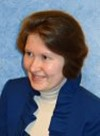### Speakers20 / 12 / 2022 - 11:20 – 12:00

## Elena A. Ivanova

Higher School of Theoretical Mechanics, Russia

#### Mechanics as the Method of Studying Nature

##### Abstract

It is known that, before the beginning of the 20th century, all physical processes were described by means of mechanical models. We use an analogous approach, but we are based on different mechanical models. The fundamental differences between our models and the models of the 19th century are as follows. Firstly, our models include rotational degrees of freedom. Secondly, our models are based on using open systems. We consider mechanics to be the method of studying physical process. This method consists of the following steps. 1) We choose a mechanical model corresponding to our intuitive understanding of the nature of the physical process. 2) We formulate differential equations describing the mechanical model. 3) We suggest analogies between mechanical quantities and quantities characterizing the physical process. 4) We rewrite the differential equations in terms of the physical quantities. 5) We make sure that, in some particular cases, the obtained equations coincide with the known physical equations. 6) We interpret the exact differential equations as a generalization of the known physical equations. We emphasize that the purpose of our study is not to derive known physical equations, but to obtain new physical equations that generalize the known equations. We present two models that allow us to obtain generalizations of Maxwell's equations. One of these models allows us to obtain equations that are a three-dimensional analogue of Kirchhoff’s laws for electrical circuits. Another model allows us to generalize Maxwell’s equations to the case of permanent magnets.